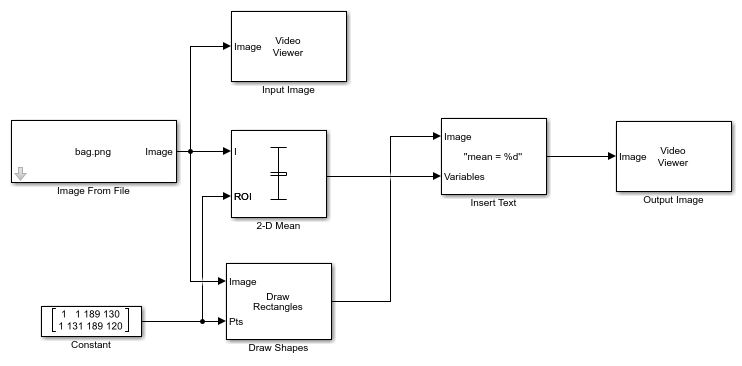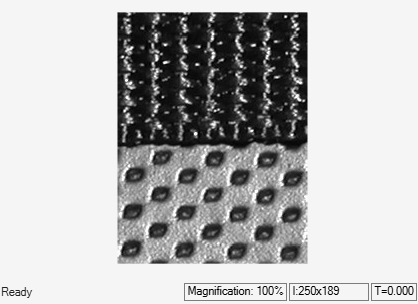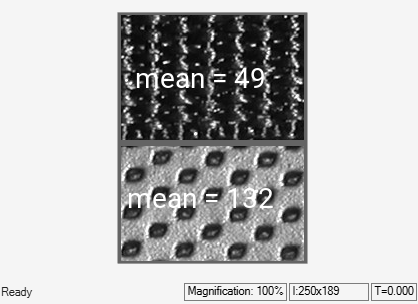# Compute Mean of ROIs in Image

This example shows how to compute the mean of regions of interest (ROIs) in an input image.

### Example Model

```modelname = 'ex_blk2dmean.slx'; open_system(modelname) ```The model reads an input image using the Image From File source block. The input image in the example has two different texture regions. To compute the mean of these regions of interest, the model uses a 2-D Mean block with these parameters:

• Running mean: `off`

• Find the mean value over: `Entire input`

• Enable ROI processing: `Rectangles`

• ROI portion to process: `Entire ROI`

• Output: `Individual statistics for each ROI`

The Constant block specifies the coordinates and dimensions for two rectangular ROIs. The Draw Shapes block overlays the bounding boxes for each rectangular ROI onto the output image. The model also displays the computed mean values in the output image using an Insert Text block.

### Simulate and Display Results

Run the model. The model displays both the input image and output image using Video Viewer blocks. Verify that the ROI around the darker region of the image shows a lower mean value than the ROI around the brighter region. The lower mean value indicates that the darker region has a lower average intensity value than the brighter region.

```sim(modelname); ```